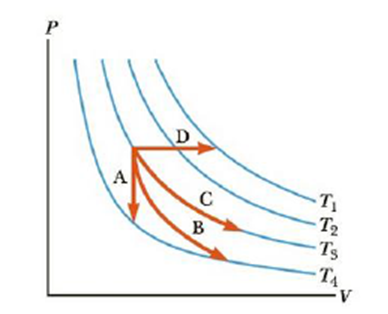Chapter 12.3, Problem 12.2QQ

Chapter
Section
Textbook Problem

Identify the paths A, B, C, and D in Figure 12.11 as isobaric, isothermal, isovolumetric, or adiabatic. For path B, Q = 0.Figure 12.11 (Quick Quiz 12.2) Identify the nature of paths A, B, C, and D

To determine
The thermal process for paths A, B, C and D.

Explanation

The paths A, B, C and D can be found using the study of thermal process.

The path A in the figure shows an isovolumetric process because it proceeds at constant volume. Thus, it will be a vertical diagram. Hence, path A is referred as isovolumetric.

For path B, Q=0 . Therefore, it is an adiabatic process. An adiabatic process is a process in which no energy enters or leaves the system by heat. Thus, Q will be zero. Hence, path B is referred as adiabatic.

In the path C, the temperature is constant

Still sussing out bartleby?

Check out a sample textbook solution.

See a sample solution

The Solution to Your Study Problems

Bartleby provides explanations to thousands of textbook problems written by our experts, many with advanced degrees!

Get Started

Why are interstellar lines so narrow?

Horizons: Exploring the Universe (MindTap Course List)

Name the following alkanes.

Chemistry: Principles and Reactions

What impact has recombinant DNA technology had on genetics and society?

Human Heredity: Principles and Issues (MindTap Course List)

What is density stratification?

Oceanography: An Invitation To Marine Science, Loose-leaf Versin